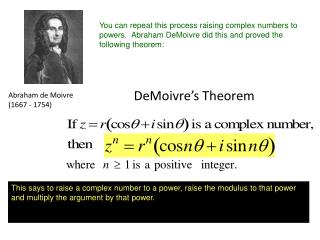DownloadDownload PresentationAbraham de Moivre (1667 - 1754)

# Abraham de Moivre (1667 - 1754)

Download Presentation## Abraham de Moivre (1667 - 1754)

- - - - - - - - - - - - - - - - - - - - - - - - - - - E N D - - - - - - - - - - - - - - - - - - - - - - - - - - -
##### Presentation Transcript

1. You can repeat this process raising complex numbers to powers. Abraham DeMoivre did this and proved the following theorem: Abraham de Moivre(1667 - 1754) DeMoivre’s Theorem This says to raise a complex number to a power, raise the modulus to that power and multiply the argument by that power.

2. but in Quad II This theorem is used to raise complex numbers to powers. It would be a lot of work to find you would need to FOIL and multiply all of these together and simplify powers of i --- UGH! Instead let's convert to polar form and use DeMoivre's Theorem.

3. Solve the following over the set of complex numbers: We know that if we cube root both sides we could get 1 but we know that there are 3 roots. So we want the complex cube roots of 1. Using DeMoivre's Theorem with the power being a rational exponent (and therefore meaning a root), we can develop a method for finding complex roots. This leads to the following formula:

4. Let's try this on our problem. We want the cube roots of 1. We want cube root so our n = 3. Can you convert 1 to polar form? (hint: 1 = 1 + 0i) We want cube root so use 3 numbers here Once we build the formula, we use it first with k = 0 and get one root, then with k = 1 to get the second root and finally with k = 2 for last root.

5. Here's the root we already knew. If you cube any of these numbers you get 1. (Try it and see!)

6. We found the cube roots of 1 were: Let's plot these on the complex plane about 0.9 each line is 1/2 unit Notice each of the complex roots has the same magnitude (1). Also the three points are evenly spaced on a circle. This will always be true of complex roots.

7. Acknowledgement I wish to thank Shawna Haider from Salt Lake Community College, Utah USA for her hard work in creating this PowerPoint. www.slcc.edu Shawna has kindly given permission for this resource to be downloaded from www.mathxtc.com and for it to be modified to suit the Western Australian Mathematics Curriculum. Stephen Corcoran Head of Mathematics St Stephen’s School – Carramar www.ststephens.wa.edu.au